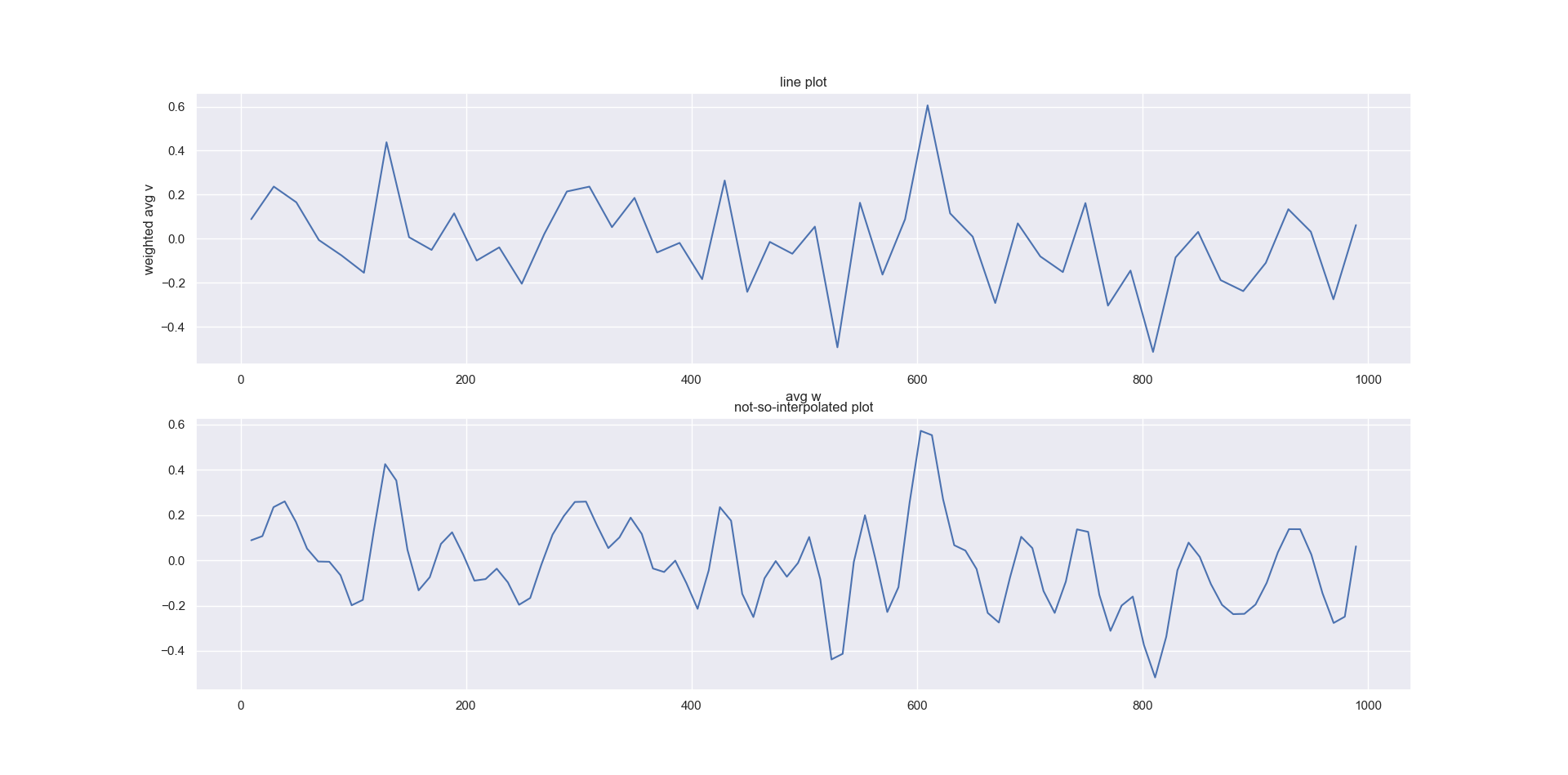# #StackBounty: #python #pandas #matplotlib #pandas-groupby #weighted-average How to smooth and plot x vs weighted average of y, weighted…

### Bounty: 150

I have a dataframe with a column of weights and one of values. I’d need:

• to discretise weights and, for each interval of weights, plot the
weighted average of values
, then
• to extend the same logic to another
variable: discretise z, and for each interval, plot the weighted
average of values, weighted by weights

Is there an easy way to achieve this?I have found a way, but it seems a bit cumbersome:

• I discretise the dataframe with pandas.cut()
• do a groupby and calculate the weighted average
• plot the mean of each bin vs the weighted average
• I have also tried to smooth the curve with a spline, but it doesn’t do much

Basically I’m looking for a better way to produce a more smoothed curve.

My output looks like this:and my code, with some random data, is:

``````import numpy as np
import pandas as pd
import matplotlib.pyplot as plt
import seaborn as sns
from scipy.interpolate import make_interp_spline, BSpline

n=int(1e3)
df=pd.DataFrame()
np.random.seed(10)
df['w']=np.arange(0,n)
df['v']=np.random.randn(n)
df['ranges']=pd.cut(df.w, bins=50)
df['one']=1.
def func(x, df):
# func() gets called within a lambda function; x is the row, df is the entire table
b1= x['one'].sum()
b2 = x['w'].mean()
b3 = x['v'].mean()
b4=( x['w'] * x['v']).sum() / x['w'].sum() if x['w'].sum() >0 else np.nan

cols=['# items','avg w','avg v','weighted avg v']
return pd.Series( [b1, b2, b3, b4], index=cols )

summary = df.groupby('ranges').apply(lambda x: func(x,df))

sns.set(style='darkgrid')

fig,ax=plt.subplots(2)
sns.lineplot(summary['avg w'], summary['weighted avg v'], ax=ax)
ax.set_title('line plot')

xnew = np.linspace(summary['avg w'].min(), summary['avg w'].max(),100)
spl = make_interp_spline(summary['avg w'], summary['weighted avg v'], k=5) #BSpline object
power_smooth = spl(xnew)
sns.lineplot(xnew, power_smooth, ax=ax)
ax.set_title('not-so-interpolated plot')
``````

Get this bounty!!!

This site uses Akismet to reduce spam. Learn how your comment data is processed.